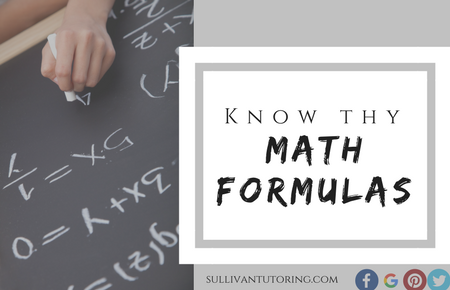# Know thy SAT & ACT math formulas#### By Scott Sullivan  |  President and Founder, Sullivan Tutoring

Test-taking is largely about confidence. One way to build that confidence on the SAT and ACT math is by memorizing necessary formulas.

The SAT provides 15 math formulas at the beginning of each math section. By our count, there are 30 other formulas you should aim to memorize for the SAT. Whereas many of the basic ones –  like Pythagorean Theorem, the 45-45-90 and 30-60-90 triangles and the main area formulas – are given, most of the crucial ones are not, including the two main slope formulas, the three forms of a parabola and the circle formula in the xy plane.

The ACT provides no formulas at the beginning of each section. By our count, there are 58 formulas you should memorize for the ACT. (These include 27 of the 30 you need to know for the SAT, 12 of the 15 which are already given on the SAT and 19 additional formulas.) You will know some of the formulas already, but make sure to learn the ones you don’t know.

The good news is that we have organized all formulas for both the SAT and ACT on the last two pages of your math SullivanCards. After determining which formulas you need to learn, review each corresponding SullivanCard for more detailed information. Even if you are unable to learn all of them, reviewing them should help your confidence and scores on test day.